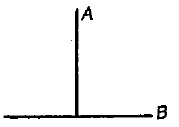A circular platform is mounted a frictionless vertical axle. Its radius R = 2m and moment of inertia about the axle are 200 ${\mathrm{kgm}}^{2}$. It is initially at rest. A 50 kg man on the edge of the platform and begins to walk along the edge at the speed of 1 $m{s}^{-1}$ relative to the ground. Time taken by the man to complete one revolution is

1.  $\mathrm{\pi s}$

2.  $\frac{3\mathrm{\pi }}{2}s$

3.  $2\mathrm{\pi s}$

4.  $\frac{\mathrm{\pi }}{2}\mathrm{s}$

Concept Questions :-

Angular momentum
High Yielding Test Series + Question Bank - NEET 2020

Difficulty Level:

The torque of force $F=-3\stackrel{^}{i}+\stackrel{^}{j}+5\stackrel{^}{k}$ acting on a point $r=7\stackrel{^}{i}+3\stackrel{^}{j}+\stackrel{^}{k}$ about origin will be

(1) $14\stackrel{^}{i}-38\stackrel{^}{j}+16\stackrel{^}{k}$              (2) $4\stackrel{^}{i}+4\stackrel{^}{j}+6\stackrel{^}{k}$

(3) $-14\stackrel{^}{i}+38\stackrel{^}{j}-16\stackrel{^}{k}$           (4) $-21\stackrel{^}{i}+3\stackrel{^}{j}+5\stackrel{^}{k}$

High Yielding Test Series + Question Bank - NEET 2020

Difficulty Level:

The motor of an engine is rotating about its axis with an angular velocity of 100 rpm.  It comes to rest is 15 s, after being switched off.  Assuming constant angular deceleration.  What are the numbers of revolutions made by it before coming to rest?

(1) 12.5          (2) 40             (3) 32.6             (4) 15.6

High Yielding Test Series + Question Bank - NEET 2020

Difficulty Level:

High Yielding Test Series + Question Bank - NEET 2020

Difficulty Level:

A T joint is formed by two identical rods A and B each of mass m and length L in the XY plane as shown. Its moment of inertia about axis passing through A and perpendicular to the plane of the joint,1.  $\frac{2{\mathrm{mL}}^{2}}{3}$

2.  $\frac{{\mathrm{mL}}^{2}}{12}$

3.  $\frac{{\mathrm{mL}}^{2}}{6}$

4.  None of these

Concept Questions :-

Moment of inertia
High Yielding Test Series + Question Bank - NEET 2020

Difficulty Level:

The moment of inertia of a loop of radius R and mass M, about any tangent line in its plane will be

(1) $\frac{3M{R}^{2}}{2}$

(2) $\frac{M{R}^{2}}{2}$

(3) $M{R}^{2}$

(4) $\frac{M{R}^{2}}{4}$

Concept Questions :-

Moment of inertia
High Yielding Test Series + Question Bank - NEET 2020

Difficulty Level:

A hollow sphere of diameter 0.2 m and mass 2 kg is rolling on an inclined plane with velocity v = 0.5 m/ s. The kinetic energy of the sphere is

1.  0.1 J

2.  0.3 J

3.  0.5 J

4.  0.42 J

Concept Questions :-

Rolling motion
High Yielding Test Series + Question Bank - NEET 2020

Difficulty Level:

A man of the mass M stands at one end of a plank of length L which lies at rest on a frictionless surfacé. The man walks to the other end of the plank. If the mass of the plank is $\frac{\mathrm{M}}{3}$, the distance that the man moves relative to the ground is

1.  $\frac{3\mathrm{L}}{4}$

2.  $\frac{\mathrm{L}}{4}$

3.  $\frac{4\mathrm{L}}{5}$

4.  $\frac{\mathrm{L}}{3}$

Concept Questions :-

Center of mass
High Yielding Test Series + Question Bank - NEET 2020

Difficulty Level:

Ratio of total kinetic energy and rotational kinetic energy in the motion of a disc is

(1) 1:1

(2) 2:7

(3) 1:2

(4) 3:1

Concept Questions :-

Rolling motion
High Yielding Test Series + Question Bank - NEET 2020

Difficulty Level:

A bomb of mass 9 kg explodes into two pieces of mass 3 kg and 6 kg. The velocity of 3 kg mass is 16 m/s. The velocity of 6 kg mass is

(1) 4 m/s

(2) 8 m/s

(3) 16 m/s

(4) 32 m/s

Concept Questions :-

Linear momentum